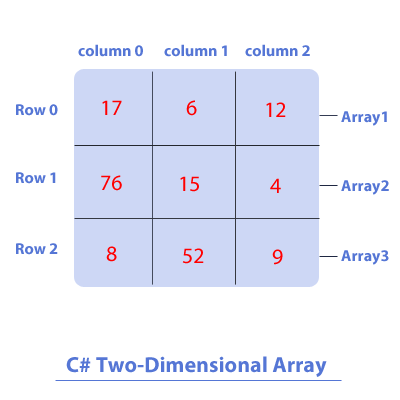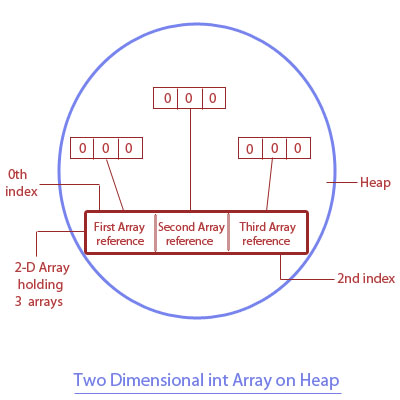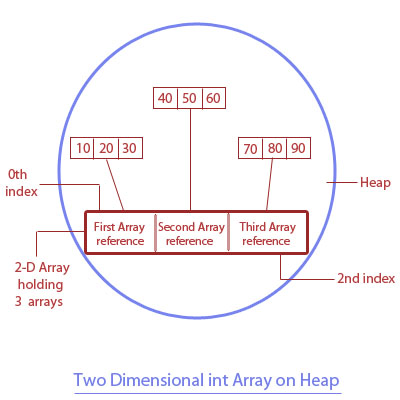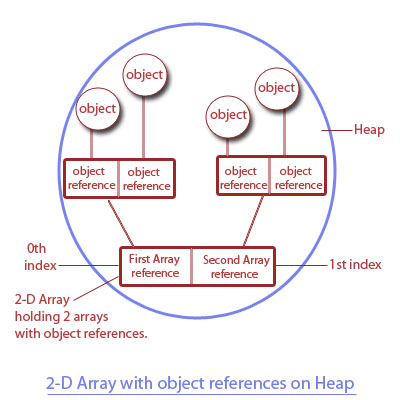Next >

# C# two-dimensional Array

A two-dimensional(2D) array is used to contain multiple 1D arrays which are holding values of the same type. The multiple 1D arrays contained in a 2D array are arranged in rows and columns i.e. each 1D array is arranged in a separate row and each element of an individual 1D array is accessed by using two index values/dimensions:
• First index/dimension represents a specific row of the element.
• Second index/dimension represents a specific column of the element.

Let us show you a pictorial representation of a 2D array.A two-dimensional array contains arrays that are holding -
• Primitive values of the same type, or
• Object references of the same type.

We will understand a two-dimensional(2D)array in three separate parts -
• Declaring a 2D array.
• Constructing a 2D array.
• Initializing a 2D array.

## Note:

Like a 1D array, 2D Array is also an object stored on the Heap memory.

## Declaration of a two-dimensional array

The general form of only declaring a two-dimensional array is :

``type[,] array-name;``
where:
• type is the type of elements this 2D array will hold, followed by a pair of square brackets with a single comma in it [ , ]. This comma is signifying that we are declaring a 2D array and it is separating the two dimensions of it.
• array-name, is the name of the array.

Let us show you an example of declaring a two-dimensional array:
``````//C# Example of two-dimensional array

using System;

class A
{
public static void Main()
{
//Declaration of a 2-D array, which will hold arrays of int values.
int[,] twoDA1;

//Declaring a 2-D array holding arrays of object references of the type class A.
A[,] twoDA2;
}
}``````

Note: So far, 2D arrays are only declared, hence neither of these 2D arrays(twoDA1 and twoDA2) exist on the heap yet, and they don't contain any values.

## Construction of a two-dimensional array

The general form of declaring and constructing a two-dimensional array is :

``type[,] array-name = new type[first-dimension , second-dimension]``
where:
• type is the type of elements this array will hold, followed by a pair of square brackets with a single comma in it [ , ]. This comma is signifying that we are declaring a 2D array.
• array-name, is the name of the array.
• new is the keyword using which an array is constructed and gets the memory allocation.
• first-dimension represents the total number of arrays contained in this 2D array.
• second-dimension represents the total number of elements in each array contained in this 2D array.

An Example of constructing a two-dimensional array.
``````//C# Example of constructing a two-dimensional array

using System;

class A
{
public static void Main()
{
//A 2D Array is constructed on the heap with a size to hold 3 int arrays.
//Where each array has 3 elements in it.
int[,] twoDA1= new int[3,3];
}
}``````

Note: If a 2D array that contains arrays with numeric primitive values is not initialized with values, each of these arrays is automatically initialized with zero values.

Post the construction of such 2D array, its impact on the heap memory(where arrays are stored) is depicted in the diagram below.As you may see in the diagram depicting the heap memory(post construction of a 2D array) -
• A 2D array in the memory is constructed, which will hold 3 int arrays.
• At the 0th index of this 2D array is the reference to the first int array.
• At the 1st index of this 2D array is the reference to the second int array.
• At the 2nd index of this 2D array is the reference to the third int array.

As these 3 int arrays are only constructed, and we have not manually initialized their elements yet, therefore, they are initialized with their default values:
• An array holding any numeric primitive type values will be automatically initialized to zero(0), and,
• An array holding object references will be automatically initialized to null.
Please look at the table to know more -

Array type Default value
char '\u0000'
byte 0
short 0
int 0
long 0
float 0
double 0
bool false
String null
Object null

## Initialization of a two-dimensional array

Our 2D int array created in the last example contains 3 arrays, so let's initialize each of the three arrays with int values. To do this, we will access each of these three int arrays using their index position in the 2D array, where:
• The reference to the first array is specified by its first-dimension index i.e. 0.
• The reference to the second array is specified by its index second-dimension index i.e. 1.
• The reference to the third array is specified by its first-dimension index i.e. 2.

Note: The second-dimension of a 2D array refers to the index position of an element in a specific array, which is represented by the first-dimension index.

``````//C# Example of initialization of a two-dimensional array

using System;

class A
{
public static void Main()
{
//A 2D array holding 3 int arrays(each array holding 3 int values).
int[,] twoDA= new int[3,3];

twoDA[0,0]=10; //inserting 10 in the first(0th index) array at its 0th index
twoDA[0,1]=20; //inserting 20 in the first(0th index) array at its 1st index
twoDA[0,2]=30; //inserting 30 in the first(0th index) array at its 2nd index

twoDA[1,0]=40; //inserting 40 in the second(1st index) array at its 0th index
twoDA[1,1]=50; //inserting 50 in the second(1st index) array at its 1st index
twoDA[1,2]=60; //inserting 60 in the second(1st index) array at its 2nd index

twoDA[2,0]=70; //inserting 70 in the third(2nd index) array at its 0th index
twoDA[2,1]=80; //inserting 80 in the third(2nd index) array at its 1st index
twoDA[2,2]=90; //inserting 90 in the third(2nd index) array at its 2nd index
}
}
``````

Depiction of the effect of the code above in the heap memory(where arrays are stored).Now let's see how a 2D array is initialized with values -

• ``twoDA[0,0]=10;``
The index of the first array in our 2D array is specified by the first-dimension i.e. zero, followed by a comma, followed by the index position in the first array where we are inserting a value, specified by the second-dimension i.e. zero, which specifies the 0th index of the first array at which we are inserting an int value 10.

• ``twoDA[0,1]=20;``
We are inserting an int value(20) in the first array at its 1st index.

• ``twoDA[0,2]=30;``
We are inserting an int value(20) in the first array at its 2nd index.

• ``twoDA[1,0]=40;``
We are inserting an int value(40) in the second array at its 0th index.

• ``twoDA[1,1]=50;``
We are inserting an int value(50) in the second array at its 1st index.

• ``twoDA[1,2]=60;``
We are inserting an int value(60) in the second array at its 2nd index.

## Reading a 2-D array holding arrays of primitive values.

We can read any element of an array contained in a 2D array using its index position in the array. For example, to read and display an element at the 0th index of 1st array in our 2D array, is simply -

``Console.WriteLine(twoDA[1,0]);	//Reading the first(1st index) array and its zeroth(0th index) element``

Just like we read the elements of a 1D array by using a single for-loop, to read all the elements of a 2D array with ease, we will use 2 for-loops, one inside another i.e. nested -
• Outer for-loop tracks through all the arrays contained in a 2D array, starting with an array at index 0 and then with the second array at index 1.
• Inner for-loop tracks through all the elements in the current array.

``````//C# Example of reading a 2-D array holding arrays of primitive values.

using System;

class A
{
public static void Main()
{
//Constructing a 2-D array holding 3 int arrays(each array holding 3 int values).
int[,] twoDA= new int[3,3];

twoDA[0,0]=10; //inserting 10 in the first(0th index) array at its 0th index
twoDA[0,1]=20; //inserting 20 in the first(0th index) array at its 1st index
twoDA[0,2]=30; //inserting 30 in the first(0th index) array at its 2nd index

twoDA[1,0]=40; //inserting 40 in the second(1st index) array at its 0th index
twoDA[1,1]=50; //inserting 50 in the second(1st index) array at its 1st index
twoDA[1,2]=60; //inserting 60 in the second(1st index) array at its 2nd index

twoDA[2,0]=70; //inserting 70 in the third(2nd index) array at its 0th index
twoDA[2,1]=80; //inserting 80 in the third(2nd index)  array at its 1st index
twoDA[2,2]=90; //inserting 90 in the third(2nd index)  array at its 2nd index

//Checking the total numbers of elements in our 2D array
//By using the Length property of array
Console.WriteLine("Length of our 2D array is : " + twoDA.Length);
Console.WriteLine("Contents of this array are : ");

//Reading our 2D array by using nested for-loops
for(int i=0; i<3; i++)
{
for(int j=0; j<3;j++)
{
Console.WriteLine(twoDA[i,j]);
}
}
}

}```
```

## Output-

``````Length of this array is : 9
Contents of this array are :
10
20
30
40
50
60
70
80
90```
```

## 2-D Array holding arrays of object references

A two-dimensional array may also hold arrays that are holding object references of the same type. Let's see a coding example -

``````//C# 2-D Array holding arrays of object references

using System;

class A
{
public static void Main()
{
//Constructing a 2D array holding 2 arrays(each array holding 2 references to objects of type A).
A[,] twoDA= new A[2,2];

twoDA[0,0]= new A(); //inserting an object of A in the first(0th index) array at its 0th index.
twoDA[0,1]= new A(); //inserting ab object of A in the first(0th index) array at its 1st index.

twoDA[1,0]= new A(); //inserting an object of A in the second(1st index) array at its 0th index.
twoDA[1,1]= new A(); //inserting an object of A in the second(1st index) array at its 1st index

//Getting the total length(total number of arrays in a 2D array).
Console.WriteLine("Number of arrays in this 2-D array : " + twoDA.Length);

//Reading the elements of a 2D array by using nested for loops
for(int i=0; i<2; i++)
{
for(int j=0; j<	2;j++)
{
Console.WriteLine(twoDA[i,j]);
}
}
}
}``````

## Output-

``````Number of arrays in this 2-D array : 4
A
A
A
A``````

Depicting the effect of the above mentioned code on the heap memory(where arrays are stored).## Program Analysis

Over here, we have created a 2D array to hold 2 arrays, with each array holding 2 references to objects of type A.
• At the zero index of a 2D array, a reference to our first array is stored. This first array contains 2 references to the objects of type A.
• At the first index of a 2D array, a reference to our second array is stored. This second array contains 2 references to the objects of type A.

## A 2D array holding object references will be automatically initialized to null

``````//C# A 2D array holding object references will be automatically initialized to null

using System;

class A
{
public static void Main()
{
//Constructing a 2D array holding 2 arrays(each array holding 2 references to objects of type A).
A[,] twoDA= new A[2,2];

//Getting the total length(total number of arrays in a 2D array).
Console.WriteLine("Number of arrays in this 2-D array : " + twoDA.Length);

//Reading the elements of a 2D array by using nested for loops
for(int i=0; i<2; i++)
{
for(int j=0; j<2;j++)
{
Console.WriteLine(twoDA[i,j] + "A null element");
}
}
}
}``````

## Output:

``````A null element
A null element
A null element
A null element``````

As you can see in the output of our last example, a 2D array to hold 4 objects of type A is constructed on the heap and its elements are not manually initialized by us, therefore, the elements of this array will be automatically initialized to null, which means that each reference in this 2D array points to no value i.e. because object was not initialized, and therefore, nothing is printed when the null is printed.